# Interval¶

class Interval(*args)

Numerical interval.

Available constructors:

Interval(dim=0)

Interval(lowerBound, upperBound, finiteLowerBound=[True]*dim, finiteUpperBound=[True]*dim)

Parameters:
dimint,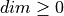Dimension of the interval. If only dim is mentioned, it leads to create the finite interval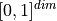. By default, an empty interval is created.

lowerBound, upperBoundfloat or sequence of float of dimension dim

Define an interval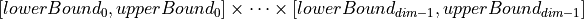. It is allowed to have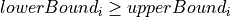for some: it simply defines an empty interval. The lowerBound and the upperBound must be of the same type. If finiteLowerBound and finiteUpperBound are mentioned, they must be sequences.

finiteLowerBoundsequence of bool of dimension dim

Flags telling for each component of the lower bound whether it is finite or not.

finiteUpperBoundsequence of bool of dimension dim

Flags telling for each component of the upper bound whether it is finite or not.

Notes

The meaning of a flag is: if flagis True, the corresponding component of the given bound is finite and its value is given by bound. If not, the corresponding component is infinite and its value is either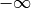if bound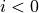or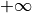if bound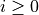.

It is possible to add or subtract two intervals and multiply an interval by a scalar.

Examples

>>> import openturns as ot
>>> # A finite interval
>>> print(ot.Interval([2.0, 3.0], [4.0, 5.0]))
[2, 4]
[3, 5]
>>> # Not finite intervals
>>> a = 2.0
>>> print(ot.Interval([a], , [True], [False]))
[2, (1) +inf[
>>> print(ot.Interval(, [a], [False], [True]))
]-inf (1), 2]
>>> # Operations with intervals:
>>> interval1 = ot.Interval([2.0, 3.0], [5.0, 8.0])
>>> interval2 = ot.Interval([1.0, 4.0], [6.0, 13.0])
>>> print(interval1 + interval2)
[3, 11]
[7, 21]
>>> # Subtraction
>>> print(interval1 - interval2)
[-4, 4]
[-10, 4]
>>> # Multiplication
>>> print(interval1 * 3)
[6, 15]
[9, 24]


Methods

 computeDistance(*args) Compute the Euclidean distance of a given point to the domain. contains(*args) Check if the given point is inside of the domain. Accessor to the object's name. Get the dimension of the domain. Tell for each component of the lower bound whether it is finite or not. Tell for each component of the upper bound whether it is finite or not. Accessor to the object's id. Get the lower bound. getMarginal(*args) Marginal accessor. Accessor to the object's name. Accessor to the object's shadowed id. Get the upper bound. Accessor to the object's visibility state. Get the volume of the interval. Test if the object is named. Test if the object has a distinguishable name. intersect(other) Get the intersection with another interval. Check if the interval is empty. Check if the interval is numerically empty. join(other) Get the smallest interval containing both the current interval and another one. Check if the given point is inside of the discretization of the interval. setFiniteLowerBound(finiteLowerBound) Tell for each component of the lower bound whether it is finite or not. setFiniteUpperBound(finiteUpperBound) Tell for each component of the upper bound whether it is finite or not. setLowerBound(lowerBound) Set the lower bound. setName(name) Accessor to the object's name. Accessor to the object's shadowed id. setUpperBound(upperBound) Set the upper bound. setVisibility(visible) Accessor to the object's visibility state.
__init__(*args)
computeDistance(*args)

Compute the Euclidean distance of a given point to the domain.

Parameters:
point or samplesequence of float or 2-d sequence of float

Point or Sample with the same dimension as the current domain’s dimension.

Returns:
distancefloat or Sample

Euclidean distance of the point to the domain.

contains(*args)

Check if the given point is inside of the domain.

Parameters:
point or samplesequence of float or 2-d sequence of float

Point or Sample with the same dimension as the current domain’s dimension.

Returns:
isInsidebool or sequence of bool

Flag telling whether the given point is inside of the domain.

getClassName()

Accessor to the object’s name.

Returns:
class_namestr

The object class name (object.__class__.__name__).

getDimension()

Get the dimension of the domain.

Returns:
dimint

Dimension of the domain.

getFiniteLowerBound()

Tell for each component of the lower bound whether it is finite or not.

Returns:
flagsBoolCollection

If the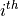element is False, the corresponding component of the lower bound is infinite. Otherwise, it is finite.

Examples

>>> import openturns as ot
>>> interval = ot.Interval([2.0, 3.0], [4.0, 5.0], [True, False], [True, True])
>>> print(interval.getFiniteLowerBound())
[1,0]

getFiniteUpperBound()

Tell for each component of the upper bound whether it is finite or not.

Returns:
flagsBoolCollection

If theelement is False, the corresponding component of the upper bound is infinite. Otherwise, it is finite.

Examples

>>> import openturns as ot
>>> interval = ot.Interval([2.0, 3.0], [4.0, 5.0], [True, False], [True, True])
>>> print(interval.getFiniteUpperBound())
[1,1]

getId()

Accessor to the object’s id.

Returns:
idint

Internal unique identifier.

getLowerBound()

Get the lower bound.

Returns:
lowerBoundPoint

Value of the lower bound.

Examples

>>> import openturns as ot
>>> interval = ot.Interval([2.0, 3.0], [4.0, 5.0], [True, False], [True, True])
>>> print(interval.getLowerBound())
[2,3]

getMarginal(*args)

Marginal accessor.

Parameters:
indexint or sequence of int

Index or indices of the selected components.

Returns:
intervalInterval

The marginal interval.

getName()

Accessor to the object’s name.

Returns:
namestr

The name of the object.

Accessor to the object’s shadowed id.

Returns:
idint

Internal unique identifier.

getUpperBound()

Get the upper bound.

Returns:
upperBoundPoint

Value of the upper bound.

Examples

>>> import openturns as ot
>>> interval = ot.Interval([2.0, 3.0], [4.0, 5.0], [True, False], [True, True])
>>> print(interval.getUpperBound())
[4,5]

getVisibility()

Accessor to the object’s visibility state.

Returns:
visiblebool

Visibility flag.

getVolume()

Get the volume of the interval.

Returns:
volumefloat

Volume contained within interval bounds.

Examples

>>> import openturns as ot
>>> interval = ot.Interval([2.0, 3.0], [4.0, 5.0], [True, False], [True, True])
>>> print(interval.getVolume())
4.0

hasName()

Test if the object is named.

Returns:
hasNamebool

True if the name is not empty.

hasVisibleName()

Test if the object has a distinguishable name.

Returns:
hasVisibleNamebool

True if the name is not empty and not the default one.

intersect(other)

Get the intersection with another interval.

Parameters:
otherIntervalInterval

Interval of the same dimension.

Returns:
intervalInterval

An interval corresponding to the intersection of the current interval with otherInterval.

Examples

>>> import openturns as ot
>>> interval1 = ot.Interval([2.0, 3.0], [5.0, 8.0])
>>> interval2 = ot.Interval([1.0, 4.0], [6.0, 13.0])
>>> print(interval1.intersect(interval2))
[2, 5]
[4, 8]

isEmpty()

Check if the interval is empty.

Returns:
isEmptybool

True if the interior of the interval is empty.

Examples

>>> import openturns as ot
>>> interval = ot.Interval([1.0, 2.0], [1.0, 2.0])
>>> interval.setFiniteLowerBound([True, False])
>>> print(interval.isEmpty())
False

isNumericallyEmpty()

Check if the interval is numerically empty.

Returns:
isEmptybool

Flag telling whether the interval is numerically empty, i.e. if its numerical volume is inferior or equal to(defined in the ResourceMap:= Domain-SmallVolume).

Examples

>>> import openturns as ot
>>> interval = ot.Interval([1.0, 2.0], [1.0, 2.0])
>>> print(interval.isNumericallyEmpty())
True

join(other)

Get the smallest interval containing both the current interval and another one.

Parameters:
otherIntervalInterval

Interval of the same dimension.

Returns:
intervalInterval

Smallest interval containing both the current interval and otherInterval.

Examples

>>> import openturns as ot
>>> interval1 = ot.Interval([2.0, 3.0], [5.0, 8.0])
>>> interval2 = ot.Interval([1.0, 4.0], [6.0, 13.0])
>>> print(interval1.join(interval2))
[1, 6]
[3, 13]

numericallyContains(point)

Check if the given point is inside of the discretization of the interval.

Parameters:
pointsequence of float

Point with the same dimension as the current domain’s dimension.

Returns:
isInsidebool

Flag telling whether the point is inside the interval bounds, not taking into account whether bounds are finite or not.

setFiniteLowerBound(finiteLowerBound)

Tell for each component of the lower bound whether it is finite or not.

Parameters:
flagssequence of bool

If theelement is False, the corresponding component of the lower bound is infinite. Otherwise, it is finite.

Examples

>>> import openturns as ot
>>> interval = ot.Interval(2)
>>> interval.setFiniteLowerBound([True, False])
>>> print(interval)
[0, 1]
]-inf (0), 1]

setFiniteUpperBound(finiteUpperBound)

Tell for each component of the upper bound whether it is finite or not.

Parameters:
flagssequence of bool

If theelement is False, the corresponding component of the upper bound is infinite. Otherwise, it is finite.

Examples

>>> import openturns as ot
>>> interval = ot.Interval(2)
>>> interval.setFiniteUpperBound([True, False])
>>> print(interval)
[0, 1]
[0, (1) +inf[

setLowerBound(lowerBound)

Set the lower bound.

Parameters:
lowerBoundsequence of float

Value of the lower bound.

Examples

>>> import openturns as ot
>>> interval = ot.Interval(2)
>>> interval.setLowerBound([-4, -5])
>>> print(interval)
[-4, 1]
[-5, 1]

setName(name)

Accessor to the object’s name.

Parameters:
namestr

The name of the object.

Accessor to the object’s shadowed id.

Parameters:
idint

Internal unique identifier.

setUpperBound(upperBound)

Set the upper bound.

Parameters:
upperBoundsequence of float

Value of the upper bound.

Examples

>>> import openturns as ot
>>> interval = ot.Interval(2)
>>> interval.setUpperBound([4, 5])
>>> print(interval)
[0, 4]
[0, 5]

setVisibility(visible)

Accessor to the object’s visibility state.

Parameters:
visiblebool

Visibility flag.

## Examples using the class¶Compute SRC indices confidence intervals

Compute SRC indices confidence intervalsFit a distribution by maximum likelihood

Fit a distribution by maximum likelihoodModel a singular multivariate distribution

Model a singular multivariate distributionEstimate a GEV on the Venice sea-levels data

Estimate a GEV on the Venice sea-levels dataBandwidth sensitivity in kernel smoothing

Bandwidth sensitivity in kernel smoothingEstimate a GEV on the Port Pirie sea-levels data

Estimate a GEV on the Port Pirie sea-levels dataEstimate a GEV on the Fremantle sea-levels data

Estimate a GEV on the Fremantle sea-levels dataEstimate a GEV on race times data

Estimate a GEV on race times dataTruncate a distribution

Truncate a distributionOverview of univariate distribution management

Overview of univariate distribution managementDistribution manipulation

Distribution manipulationCreate a customized distribution or copula

Create a customized distribution or copulaDraw minimum volume level sets

Draw minimum volume level setsExport a field to VTK

Export a field to VTKAggregate processes

Aggregate processesCreate a custom covariance model

Create a custom covariance modelUse the Box-Cox transformation

Use the Box-Cox transformationDraw a field

Draw a fieldCreate a process from random vectors and processes

Create a process from random vectors and processesTrend computation

Trend computationCreate a mesh

Create a meshCreate a linear model

Create a linear modelCompute Sobol’ indices confidence intervals

Compute Sobol' indices confidence intervalsKriging : cantilever beam model

Kriging : cantilever beam modelKriging the cantilever beam model using HMAT

Kriging the cantilever beam model using HMATExample of multi output Kriging on the fire satellite model

Example of multi output Kriging on the fire satellite modelChoose the trend basis of a kriging metamodel

Choose the trend basis of a kriging metamodelKriging with an isotropic covariance function

Kriging with an isotropic covariance functionKriging :configure the optimization solver

Kriging :configure the optimization solverValidation of a Karhunen-Loeve decomposition

Validation of a Karhunen-Loeve decompositionViscous free fall: metamodel of a field function

Viscous free fall: metamodel of a field functionMetamodel of a field function

Metamodel of a field functionUse the FORM algorithm in case of several design points

Use the FORM algorithm in case of several design pointsCreate a domain event

Create a domain eventAn illustrated example of a FORM probability estimate

An illustrated example of a FORM probability estimateCross Entropy Importance Sampling

Cross Entropy Importance SamplingCreate an event based on a process

Create an event based on a processEstimate a process-based event probability

Estimate a process-based event probabilityThe HSIC sensitivity indices: the Ishigami model

The HSIC sensitivity indices: the Ishigami modelThe PlotDesign method

The PlotDesign methodPlot Smolyak multi-indices

Plot Smolyak multi-indicesVarious design of experiments in OpenTURNS

Various design of experiments in OpenTURNSDefine a function with a field output: the viscous free fall example

Define a function with a field output: the viscous free fall exampleDefine a connection function with a field output

Define a connection function with a field outputCalibration of the Chaboche mechanical model

Calibration of the Chaboche mechanical modelSampling from an unnormalized probability density

Sampling from an unnormalized probability densityPosterior sampling using a PythonDistribution

Posterior sampling using a PythonDistributionLinear Regression with interval-censored observations

Linear Regression with interval-censored observationsOptimization with constraints

Optimization with constraintsOptimization using NLopt

Optimization using NLoptMix/max search using optimization

Mix/max search using optimizationOptimization using bonmin

Optimization using bonminQuick start guide to optimization

Quick start guide to optimizationMulti-objective optimization using Pagmo

Multi-objective optimization using PagmoOptimization of the Rastrigin test function

Optimization of the Rastrigin test functionOptimization using dlib

Optimization using dlibEfficientGlobalOptimization examples

EfficientGlobalOptimization examples